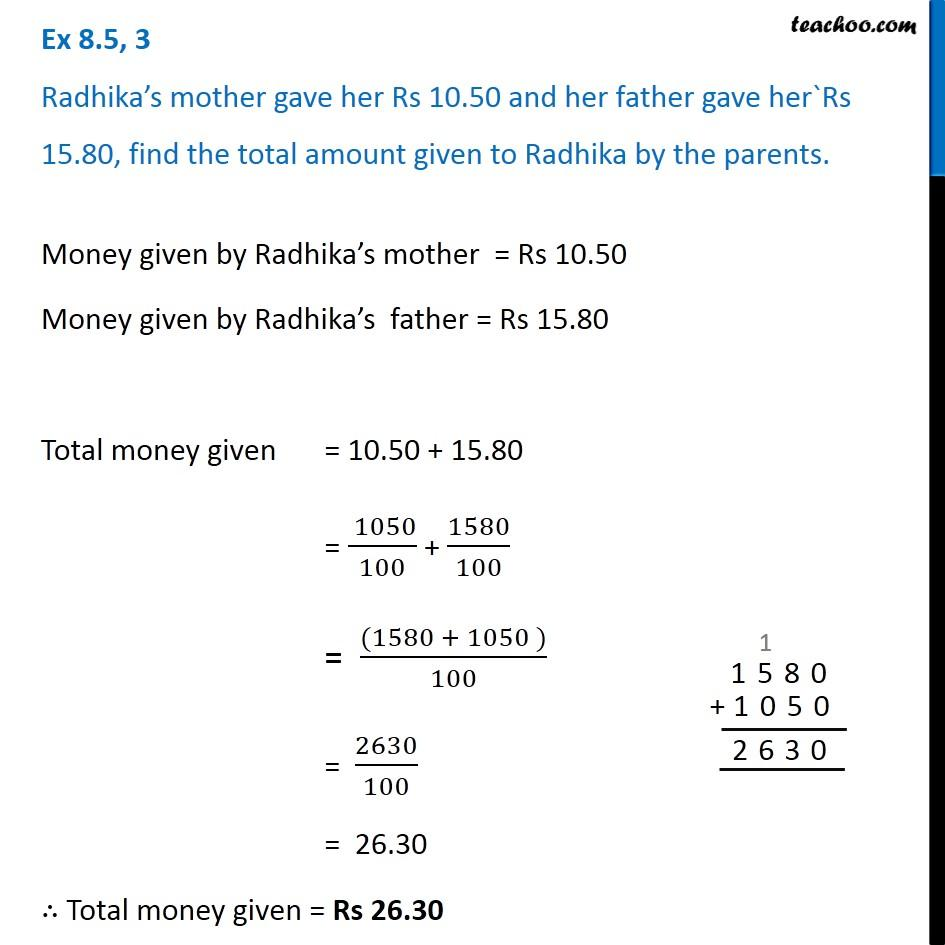Addition of decimal numbers - Statement questions

Chapter 8 Class 6 Decimals
Concept wise### Transcript

Ex 8.5, 3 Radhika s mother gave her Rs 10.50 and her father gave her`Rs 15.80, find the total amount given to Radhika by the parents.Money given by Radhika s mother = Rs 10.50 Money given by Radhika s father = Rs 15.80 Total money given = 10.50 + 15.80 = ( 1050)/100 + 1580/100 = ((1580 + 1050 ))/100 = 2630/100 = 26.30 Total money given = Rs 26.30